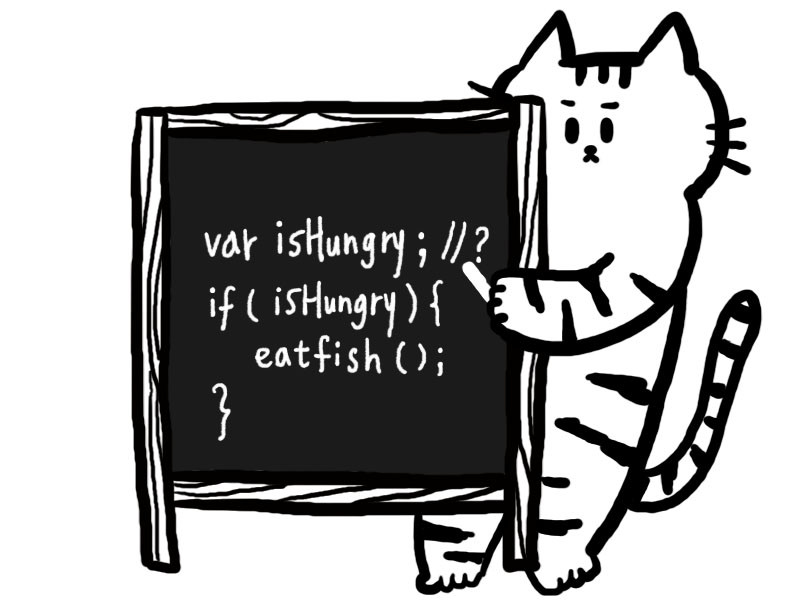DAY 10
0
Modern Web

# 計算與結果cover picture sponsor: gleammming.art

## 運算式（Expression）

• 原始型別運算式（Primitive Expressions）
``````1; // 1
``````
• 算數運算式（Arithmetic Expressions）
``````1 + 2; // 3
``````
• 字串運算式（String Expressions）
``````'Hello, ' + 'JavaScript!'; // 'Hello, JavaScript!'
``````
• 邏輯運算式（Logical Expressions）
``````5 > 1; // true
``````
• 賦值運算式（Assignment Expressions）
``````box = 360; // 360
``````

``````var one = 1;
var two = 2;
var five = 5;
var text = 'Hello, ' + 'JavaScript!';
var box;

one; // 1
one + two; // 3
text; // 'Hello, JavaScript!'
five > one; // true
box = (one + five) * two; // 12

console.log(box); // 12
``````

``````var ownMoney = 200;
var stockPrice = 300;
var haveEnough = ownMoney > stockPrice;
``````

### 真值與假值：運算式的另個含意

``````Boolean('test'); // true
Boolean(0); // false
``````

• `false`
• `0`
• `''`
• `undefined`
• `null`
• `NaN`

• `1`
• `true`
• 其他不是假值的都是真值。

• `-1`
• `' '` 包含一個空白的字串
• `'false'` 字串
• `{}` 空物件
• `[]` 空陣列

``````var ownMoney = 200
var stockPrice = 300
var haveEnough = ownMoney > stockPrice // false

if (haveEnough) {
console.log('You have enough money to pay this!')
} else {
console.log('Sorry! You need more money for this.')
}
``````

`haveEnough` 變數當中，我們透過 `ownMoney > stockPrice` 算出結果是 `false`，而對於 `if/else` 敘述句來說，條件句中的結果若是屬於 `truthy` 則會執行 `if` 語句後區塊內的程式碼；相反的，則會往後執行 `else` 語句區塊內的程式碼。

### 延伸閱讀：有副作用（Side Effects）的運算式

``````1 + 2; // 3
``````

``````var a = 1; // 1
a = 2; // 2
``````

## 算數運算子（Arithmetic Operators）

``````var box = 0;

++box; // 1

console.log(box); // 1
``````

``````var box = 0;

box++; // 0

console.log(box); // 1
``````

``````var mixedCatsColor = ['black', 'white', 'orange', 'tuxedo', 'tortoiseshell', 'tabby'];

for(var i = 0; i < mixedCatsColor.length; i++) {
console.log('There is a(n) ' + mixedCatsColor[i] + ' cat!')
}
``````

### 比較運算子（Comparison Operators）

``````// 資料來源、從 API 取得等等數值。
var wallet = 100;
var frenchFriesPrice = 60;
var hamburgerPrice = 50;
// 比較邏輯類
var setMeal = frenchFriesPrice + hamburgerPrice;
var isWalletEnoughToBuySet = wallet >= setMeal
``````

``````var wallet = 100;
var frenchFriesPrice = 60;
var hamburgerPrice = 50;
var setMeal = frenchFriesPrice + hamburgerPrice;
var isWalletEnoughToBuySet = wallet >= setMeal

if (isWalletEnoughToBuySet) {
buySetMeal()
};
``````

#### 自動轉型（coercion）

``````'100' == 100; // true => (100).toString() => '100'
``````

``````[] == [] // false
``````

``````var a = []; // 0x00 => undefined, 0x02 => []
var b = []; // 0x01 => undefined, 0x04 => []
var c = a;  // 0x02 => []

a === c // true，因為都指向 0x02 中的 []
a === b // false，指向不同的記憶體位置
c === b // false，指向不同的記憶體位置
``````

``````var stockPrice = document.querySelector('#age').value; // 從表單上取值，假設使用者填入了 100

stockPrice += 100 // 試著加上 100...

if (stockPrice > 500) {
console.log('Guess what!'); // 猜猜發生了什麼事情。
}
``````